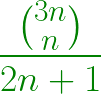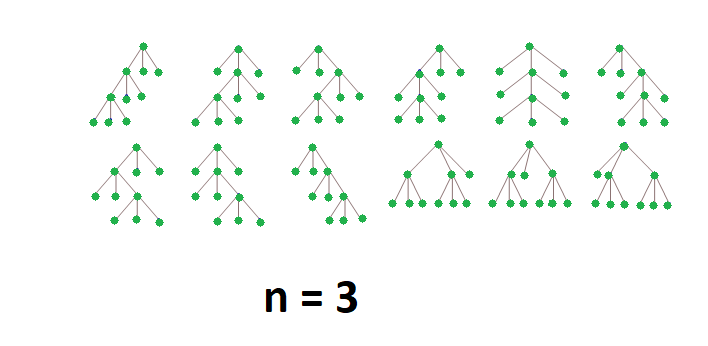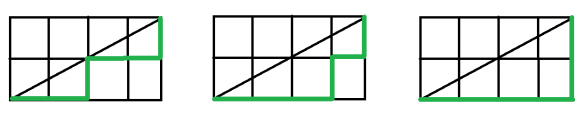Skip to content
Related Articles
Program for nth Fuss–Catalan Number
• Last Updated : 06 Apr, 2021

Fuss–Catalan Numbers are a generalization of Catalan numbers that uses triplets instead of pairs.

The Fuss-Catalan Numbers can be represented by a Series with the formula:The first few Fuss–Catalan Numbers are

1, 1, 3, 12, 55, 273, 1428, 7752, 43263, 246675………..
for n = 0, 1, 2, 3, … respectively

Applications of Fuss-Catalan number:

1. Count the number of ways to place parentheses among of 2n+1 numbers to be grouped three at a time.
Example: There are 3 ways to parenthesize {1, 2, 3, 4, 5} as triplets:
{{1, 2, 3}, 4, 5}, {1, {2, 3, 4}, 5}, {1, 2, {3, 4, 5}}

2. Count the number of complete ternary trees with n internal nodes.1.
2. Count the number of paths of length 3n through a 2n-by-n grid that does not cross above the main diagonal
Example: There are 3 paths from (0, 0) to (4, 2) that don’t cross above the diagonal:1.
2. and many more. Please refer this link for more applications

Implementation of Fuss-Catalan number:

## C++

 `// C++ program for nth Fuss–Catalan Number` `#include ``using` `namespace` `std;` `// Returns value of Binomial Coefficient C(n, k)``unsigned ``long` `int` `binomialCoeff(unsigned ``int` `n,``                                ``unsigned ``int` `k)``{``    ``unsigned ``long` `int` `res = 1;` `    ``// Since C(n, k) = C(n, n-k)``    ``if` `(k > n - k)``        ``k = n - k;` `    ``// Calculate value of``    ``//[n*(n-1)*---*(n-k+1)] / [k*(k-1)*---*1]``    ``for` `(``int` `i = 0; i < k; ++i) {``        ``res *= (n - i);``        ``res /= (i + 1);``    ``}` `    ``return` `res;``}` `// A Binomial coefficient based function``// to find nth Fuss–Catalan number in O(n) time``unsigned ``long` `int` `Fuss_catalan(unsigned ``int` `n)``{``    ``// Calculate value of 3nCn``    ``unsigned ``long` `int` `c = binomialCoeff(3 * n, n);` `    ``// return 3nCn/(2n+1)``    ``return` `c / (2 * n + 1);``}` `// Driver code``int` `main()``{``    ``for` `(``int` `i = 0; i < 10; i++)``        ``cout << Fuss_catalan(i) << ``" "``;``    ``return` `0;``}`

## Java

 `// Java program for nth Fuss-Catalan Number``class` `GFG``{` `// Returns value of Binomial Coefficient C(n, k)``static` `int` `binomialCoeff(``int` `n, ``int` `k)``{``    ``int` `res = ``1``;` `    ``// Since C(n, k) = C(n, n-k)``    ``if` `(k > n - k)``        ``k = n - k;` `    ``// Calculate value of``    ``//[n*(n-1)*---*(n-k+1)] / [k*(k-1)*---*1]``    ``for` `(``int` `i = ``0``; i < k; ++i)``    ``{``        ``res *= (n - i);``        ``res /= (i + ``1``);``    ``}``    ``return` `res;``}` `// A Binomial coefficient based function``// to find nth Fuss-Catalan number in O(n) time``static` `int` `Fuss_catalan(``int` `n)``{``    ``// Calculate value of 3nCn``    ``int` `c = binomialCoeff(``3` `* n, n);` `    ``// return 3nCn/(2n+1)``    ``return` `c / (``2` `* n + ``1``);``}` `// Driver code``public` `static` `void` `main(String []args)``{``    ``for` `(``int` `i = ``0``; i < ``10``; i++)``        ``System.out.print(Fuss_catalan(i) + ``" "``);``}``}` `// This code is contributed by 29AjayKumar`

## Python3

 `# Python3 program for nth Fuss–Catalan Number` `# Returns value of Binomial Coefficient C(n, k)``def` `binomialCoeff(n, k) :` `    ``res ``=` `1``;` `    ``# Since C(n, k) = C(n, n-k)``    ``if` `(k > n ``-` `k) :``        ``k ``=` `n ``-` `k;` `    ``# Calculate value of``    ``# [n*(n-1)*---*(n-k+1)] / [k*(k-1)*---*1]``    ``for` `i ``in` `range``(k) :``        ` `        ``res ``*``=` `(n ``-` `i);``        ``res ``/``/``=` `(i ``+` `1``);` `    ``return` `res;` `# A Binomial coefficient based function``# to find nth Fuss–Catalan number in O(n) time``def` `Fuss_catalan(n) :` `    ``# Calculate value of 3nCn``    ``c ``=` `binomialCoeff(``3` `*` `n, n);``    ` `    ``# return 3nCn/(2n+1)``    ``return` `c ``/``/` `(``2` `*` `n ``+` `1``);` `# Driver code``if` `__name__ ``=``=` `"__main__"` `:` `    ``for` `i ``in` `range``(``10``) :``        ``print``(Fuss_catalan(i), end ``=` `" "``);` `# This code is contributed by AnkitRai01`

## C#

 `// C# program for nth Fuss-Catalan Number``using` `System;` `class` `GFG``{` `// Returns value of Binomial Coefficient C(n, k)``static` `int` `binomialCoeff(``int` `n, ``int` `k)``{``    ``int` `res = 1;` `    ``// Since C(n, k) = C(n, n-k)``    ``if` `(k > n - k)``        ``k = n - k;` `    ``// Calculate value of``    ``//[n*(n-1)*---*(n-k+1)] / [k*(k-1)*---*1]``    ``for` `(``int` `i = 0; i < k; ++i)``    ``{``        ``res *= (n - i);``        ``res /= (i + 1);``    ``}``    ``return` `res;``}` `// A Binomial coefficient based function``// to find nth Fuss-Catalan number in O(n) time``static` `int` `Fuss_catalan(``int` `n)``{``    ``// Calculate value of 3nCn``    ``int` `c = binomialCoeff(3 * n, n);` `    ``// return 3nCn/(2n+1)``    ``return` `c / (2 * n + 1);``}` `// Driver code``public` `static` `void` `Main(String []args)``{``    ``for` `(``int` `i = 0; i < 10; i++)``        ``Console.Write(Fuss_catalan(i) + ``" "``);``}``}` `// This code is contributed by PrinciRaj1992`

## Javascript

 ``
Output:
`1 1 3 12 55 273 1428 7752 43263 246675`

Time Complexity: O(n)

Attention reader! Don’t stop learning now. Get hold of all the important DSA concepts with the DSA Self Paced Course at a student-friendly price and become industry ready.  To complete your preparation from learning a language to DS Algo and many more,  please refer Complete Interview Preparation Course.

In case you wish to attend live classes with industry experts, please refer Geeks Classes Live

My Personal Notes arrow_drop_up